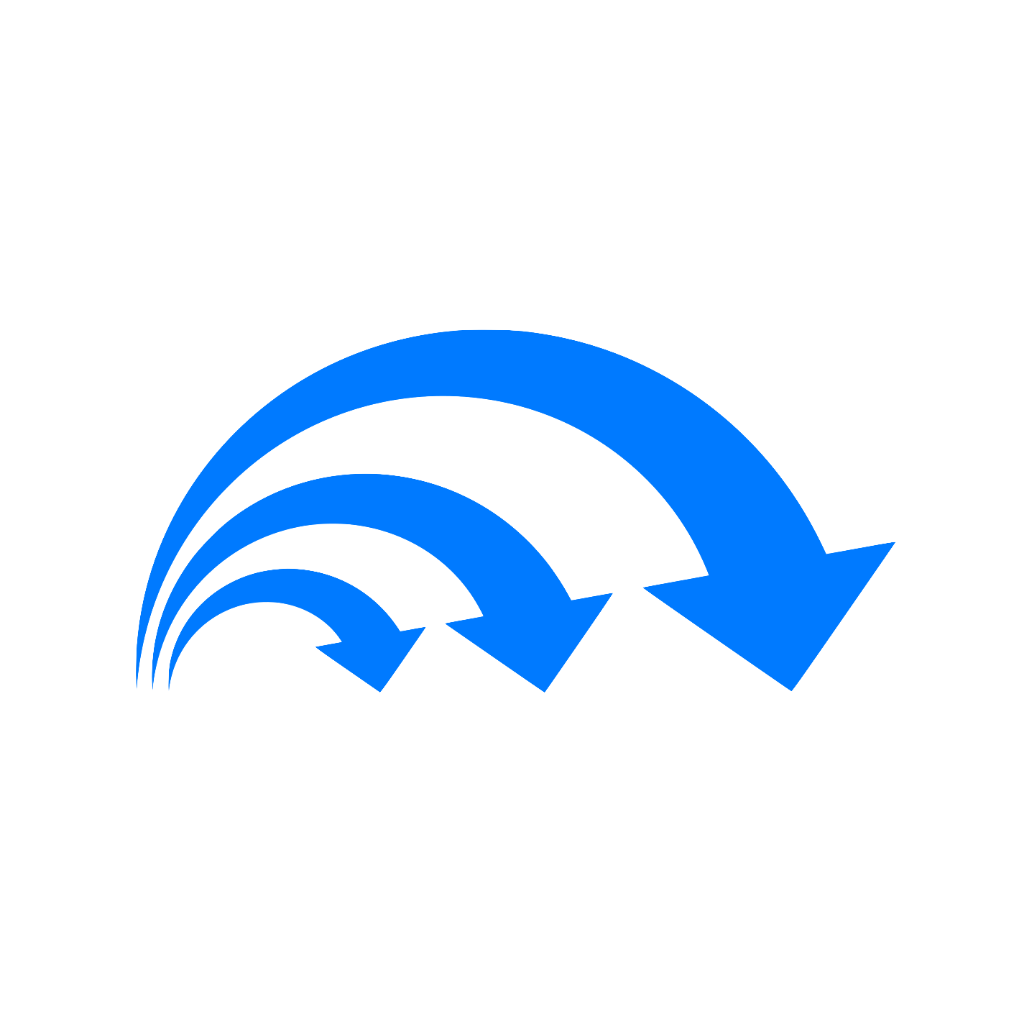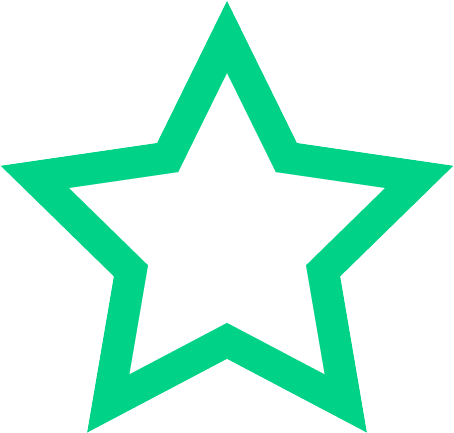# Enhanced Math 2 Properties of Special Quadrilaterals

Mihir Ranjan (lvl 10)
Parallelograms

### Preview this deck

Properties of Parallelograms

Front### 0.0

0 reviews

 5 0 4 0 3 0 2 0 1 0

Active users

1

All-time users

1

Favorites

0

Last updated

7 months ago

Date created

Mar 4, 2021

## Cards(16)

Parallelograms

(2 cards)

Properties of Parallelograms

Front
1. Opposite angles are congruent
2. Consecutive angles are supplemental
3. Diagonals bisect each other
4. Opposite sides are congruent
Back

Definition of Parallelograms

Front

A quadrilateral with two pairs of parallel sides

Back

Rectangles

(2 cards)

Properties of Rectangles

Front

1) 2 sets of parallel sides

2) Opposite angles are congruent

3) Consecutive angles are supplemental

4) Diagonals bisect each other

5) Opposite Sides are congruent

6) Diagonals are congruent

Back

Definition of Rectangles

Front

A quadrilateral with four right angles

Back

Rhombus

(2 cards)

Definition of Rhombus

Front

A quadrilateral with four congruent sides

Back

Properties of Rhombus(7)

Front

1) 2 sets of parallel sides

2) Opposite angles are congruent

3) Consecutive angles are supplemental

4) Diagonals bisect each other

5) Opposite sides are congruent

6) Diagonals are perpendicular

7) Diagonals bisect the angles of the rhombus

Back

Squares

(2 cards)

Properties of Squares

Front
1. 2 sets of parallel lines
2. Opposite angles are congruent
3. Consecutive angles are supplemental
4. Diagonals bisect each other
5. Opposite sides are congruent
6. Diagonals are congruent
7. Diagonals are perpendicular
8. Diagonals bisect the angles of the square
Back

Definition of Squares

Front

A quadrilateral with four congruent sides

Back

Kites

(2 cards)

Definition of a Kite

Front

A quadrilateral with two distinct pairs of consecutive congruent sides

Back

Properties of Kites

Front
1. Diagonals are perpendicular
2. Exactly one pair of opposite angles are congruent
3. One diagonal bisects the other
4. A diagonal bisects one pair of opposite angles
Back

Trapezoids

(2 cards)

Properties of Trapezoids

Front
1. Angles on each leg are supplemental
Back

Definition of A Trapezoid

Front

A quadrilateral with one pair of parallel sides

Back

Isosceles Trapezoids

(2 cards)

Properties if Isosceles

Front
1. Angles on each leg are supplemental
2. Base angles are congruent
3. Diagonals are congruent
Back

Definition of Isosceles Trapezoids

Front

A trapezoid in which the non-parallel sides are congruent

Back

Right Trapezoids

(2 cards)

Properties of Right Trapezoids

Front
1. Angels on each leg are supplemental
Back

Definition of Right Trapezoid

Front

A trapezoid with two right angles

Back